# Team:NNU-China/Model

NNU-China

(Gold Medal#3)

## The format conversion of model:

###### 2. Output the model of EXCEL foramt:
writeCbModel(model, 'format','xls', 'fileName', ' iECBD_1354_COBRA.xlsx')
###### 3. Input the EXCEL format model into Matlab:
model=xls2model(' iECBD_1354_COBRA.xlsx')
###### 4. Output the standard SBML format model written by COBRA toolbox
writeCbModel(model, 'format','sbml', 'fileName', 'iECBD_1354_COBRA.xml')
###### 5. Input SBML format model written by COBRA toolbox
model = importModel('iECBD_1354_COBRA.xml',false)
###### 6. Output the standard SBML format model written by RAVEN toolbo
exportToExcelFormat(model,'iECBD_1354_RAVEN.xlsx')

## The modification of basic model:

###### (5) Splitting the format of biomass equations, from one reaction into nine reactions:

Before splitting:
0.09158 cys__L[c] + 0.26316 gln__L[c] + 0.000223 pydx5p[c] + 0.13351 ctp[c] + 0.2151 gtp[c] + 0.000223 fad[c] + 0.21579 ser__L[c] + 0.056843 trp__L[c] + 0.027017 dgtp[c] + 0.15369 met__L[c] + 0.000223 thf[c] + 0.000709 cu2[c] + 0.005205 cl[c] + 0.004338 so4[c] + 0.000223 pheme[c] + 0.61264 gly[c] + 0.026166 datp[c] + 0.000122 bmocogdp[c] + 0.1441 utp[c] + 0.34316 lys__L[c] + 0.45053 leu__L[c] + 0.027017 dctp[c] + 0.017868 pe160[c] + 0.000576 coa[c] + 0.25369 thr__L[c] + 0.29579 arg__L[c] + 2.6e-05 2fe2s[c] + 0.054154 pe161[c] + 0.000691 mn2[c] + 0.045946 pe160[p] + 54.1248 atp[c] + 0.00026 4fe4s[c] + 0.007808 fe3[c] + 2e-06 btn[c] + 0.000341 zn2[c] + 0.000223 ribflv[c] + 0.006715 fe2[c] + 0.000223 sheme[c] + 0.000223 amet[c] + 0.008675 mg2[c] + 0.19519 k[c] + 0.42316 val__L[c] + 0.1379 tyr__L[c] + 0.000223 mlthf[c] + 0.000223 2ohph[c] + 2.5e-05 cobalt2[c] + 0.019456 kdo2lipid4[e] + 5.5e-05 udcpdp[c] + 0.013894 murein5px4p[p] + 0.02106 pe161[p] + 0.005205 ca2[c] + 0.000323 ni2[c] + 0.24105 asn__L[c] + 0.29053 ile__L[c] + 0.26316 glu__L[c] + 0.094738 his__L[c] + 0.013013 nh4[c] + 48.6015 h2o[c] + 0.18527 phe__L[c] + 0.22106 pro__L[c] + 0.51369 ala__L[c] + 0.026166 dttp[c] + 0.000223 10fthf[c] + 0.000223 thmpp[c] + 0.000447 nadp[c] + 7e-06 mobd[c] + 0.24105 asp__L[c] + 0.001831 nad[c] -> 0.7739 ppi[c] + 53.95 adp[c] + 53.9457 pi[c] + 53.95 h[c]

After splitting:
① Protein:
0.26316 gln__L[c] + 0.24105 asp__L[c] + 0.51369 ala__L[c] + 0.29579 arg__L[c] + 0.09158 cys__L[c] + 0.61264 gly[c] + 0.22106 pro__L[c] + 0.24105 asn__L[c] + 0.15369 met__L[c] + 0.34316 lys__L[c] + 0.21579 ser__L[c] + 0.094738 his__L[c] + 0.29053 ile__L[c] + 0.45053 leu__L[c] + 0.056843 trp__L[c] + 0.18527 phe__L[c] + 0.25369 thr__L[c] + 0.1379 tyr__L[c] + 0.42316 val__L[c] + 0.26316 glu__L[c] -> protein[c]
② DNA:
0.027017 dctp[c] + 0.026166 datp[c] + 0.027017 dgtp[c] + 0.026166 dttp[c] -> dna[c]
③ RNA:
0.1748 atp[c] + 0.1441 utp[c] + 0.13351 ctp[c] + 0.2151 gtp[c] -> rna[c]
④ Lipid:
0.019456 kdo2lipid4[e] + 0.017868 pe160[c] + 0.054154 pe161[c] + 0.045946 pe160[p] + 0.02106 pe161[p] -> lipid[c]
⑤ Carbohydrate:
0.013894 murein5px4p[p] -> carbohydrate[c]
⑥ Ion:
0.000341 zn2[c] + 0.006715 fe2[c] + 0.000709 cu2[c] + 2.6e-05 2fe2s[c] + 0.005205 ca2[c] + 2.5e-05 cobalt2[c] + 0.005205 cl[c] + 0.007808 fe3[c] + 0.19519 k[c] + 0.008675 mg2[c] + 0.000691 mn2[c] + 7e-06 mobd[c] + 0.000323 ni2[c] + 0.004338 so4[c] + 0.013013 nh4[c] + 0.00026 4fe4s[c] -> ion[c]
⑦ Cofactor:
0.001831 nad[c] + 0.000447 nadp[c] + 0.000576 coa[c] + 0.000223 amet[c] + 0.000223 fad[c] + 0.000223 10fthf[c] + 0.000223 thf[c] + 0.000223 pydx5p[c] + 0.000223 ribflv[c] + 0.000122 bmocogdp[c] + 2e-06 btn[c] + 0.000223 pheme[c] + 0.000223 mlthf[c] + 5.5e-05 udcpdp[c] + 0.000223 2ohph[c] + 0.000223 thmpp[c] + 0.000223 sheme[c] -> cofactor[c]
⑧ Biomass:
53.95 atp[c] + 48.6015 h2o[c] + protein[c] + dna[c] + rna[c] + lipid[c] + carbohydrate[c] + ion[c] + cofactor[c] -> 53.95 adp[c] + 53.9457 pi[c] + 53.95 h[c] + 0.7739 ppi[c] + biomass[c]
⑨ Growth:biomass[c] <=>

###### We first downloaded the sequence of SMAP protein from NCBI. Then the composition of 20 proteins in the sequence was count (Table 1).
Table 1. The amino acids composition of SMAP protein
Amino acid Count Amino acid Count
Alanine 12 Leucine 22
Arginine 15 Lysine 9
Asparagine 7 Methionine 1
Aspartic Acid 7 Phenylalanine 3
Cysteine 4 Proline 9
Glutamic acid 11 Serine 11
Glutamine 9 Threonine 7
Glycine 10 Typtophan 1
Histidine 1 Tyrosine 3
Isoleucine 6 Valine 12

###### Then, we get the synthetic reaction of SMAP:

34.52 atp[c] + 0.5053 glu__L[c] + 0.6176 gln__L[c] + 0.393 asp__L[c] + 0.6737 ala__L[c] + 0.8422 arg__L[c] + 0.2246 cys__L[c] + 0.5614 gly[c] + 0.5053 pro__L[c] + 0.393 asn__L[c] + 0.0561 met__L[c] + 0.5053 lys__L[c] + 0.6176 ser__L[c] + 0.0561 his__L[c] + 0.3369 ile__L[c] + 1.2352 leu__L[c] + 0.0561 trp__L[c] + 0.1684 phe__L[c] + 0.393 thr__L[c] + 0.1684 tyr__L[c] + 0.6737 val__L[c] -> 34.52 adp[c] + 34.52 pi[c] + smap[c]

## The construction of enzyme constraint mode

#### ② Construct enzyme-constraint model

###### The original E. coli BL21 model of iECBD_1354_RAVEN is read into Matlab, which involves parameters such as the number of reactions, the number of metabolites, the number of genes, etc. contained in the model (Fig. 1).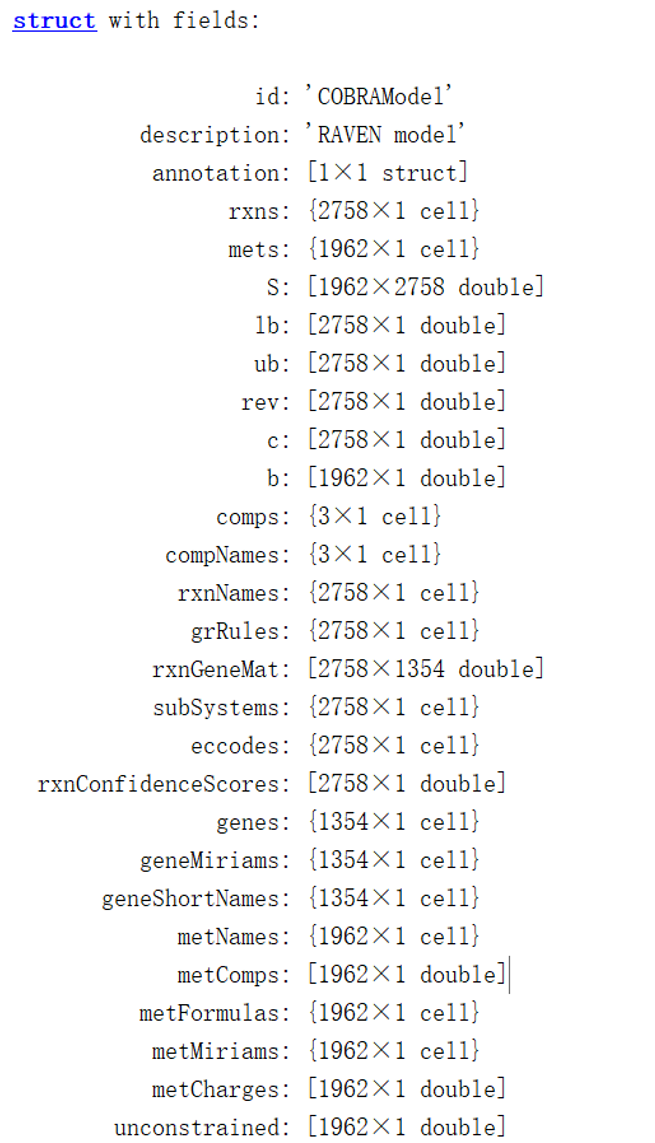Fig. 1. The original model of iECBD_1354_RAVEN

###### Matching the gene within the model with the data collected by Uniprot and KEGG database, which includes information about substrates, productions, EC codes, molecular mass etc. that are involved in biochemical reactions (Fig. 2)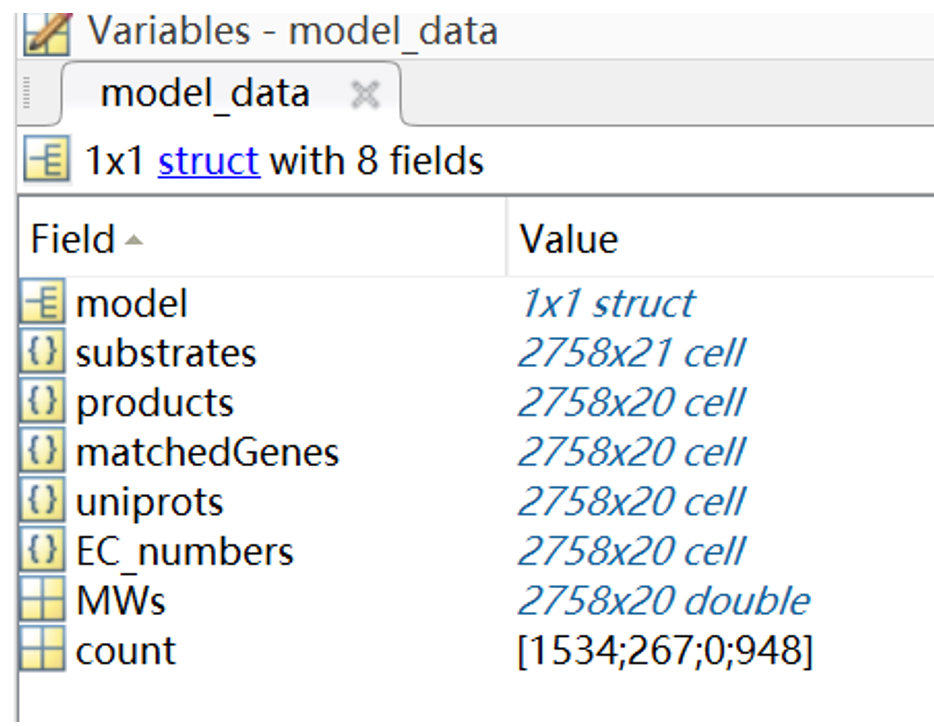Fig. 2. The enzyme data generated by Uniprot and KEGG

###### Using this command, the extraction of kcat number from the collection of data is achieved (Fig. 3).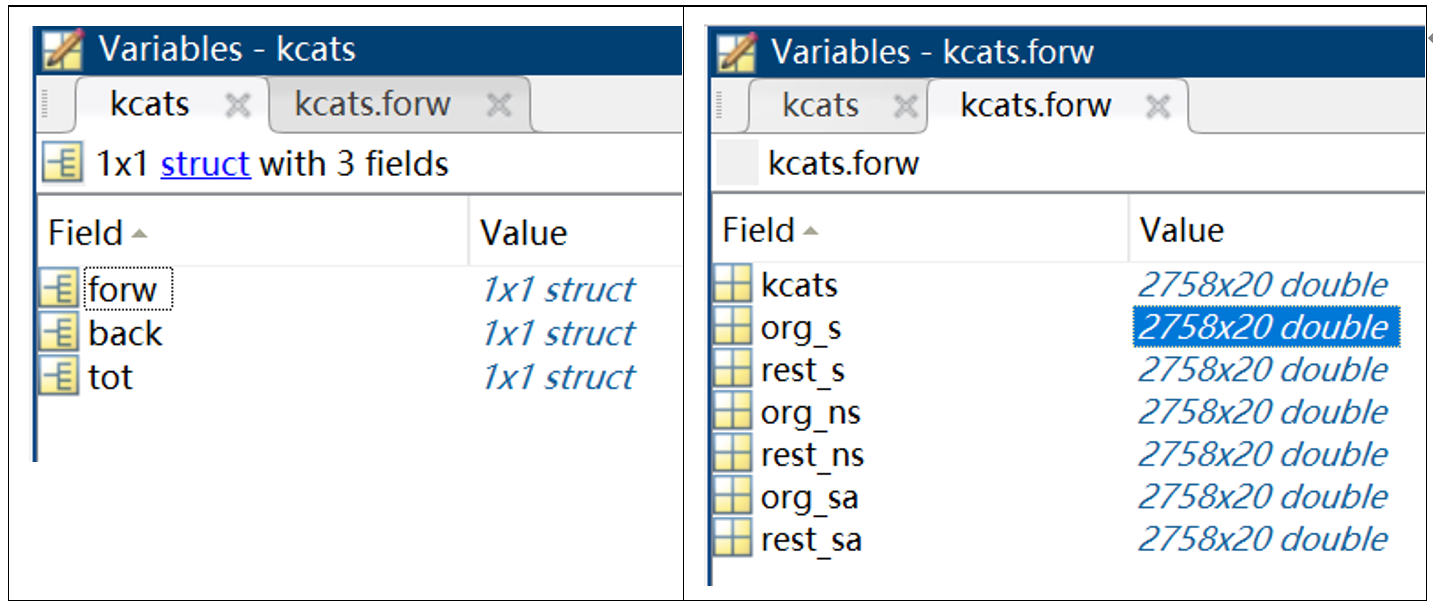Fig. 3. The matched enzyme properties of E. coli. A: total matched results. B: detailed information about matched enzyme.N

###### This command achieving the match of kcat number with the model (Fig. 4)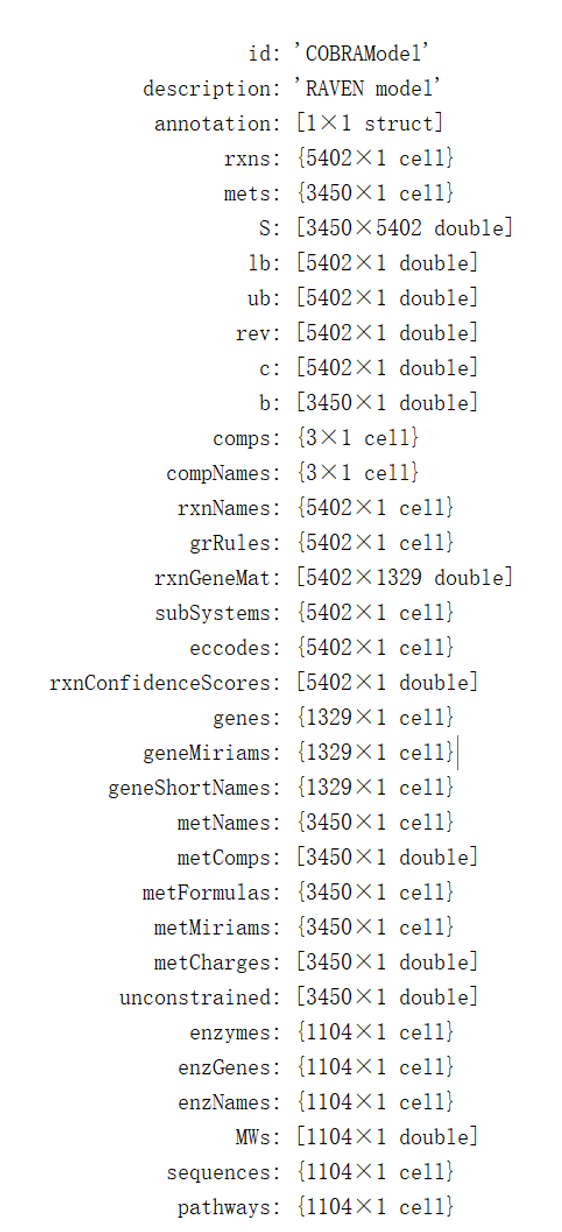Fig. 4. Structure of enzyme-constrained model

###### Modifying the model by operating this command, and the results shows that a series of duplicate reactions have been removed (Fig. 5).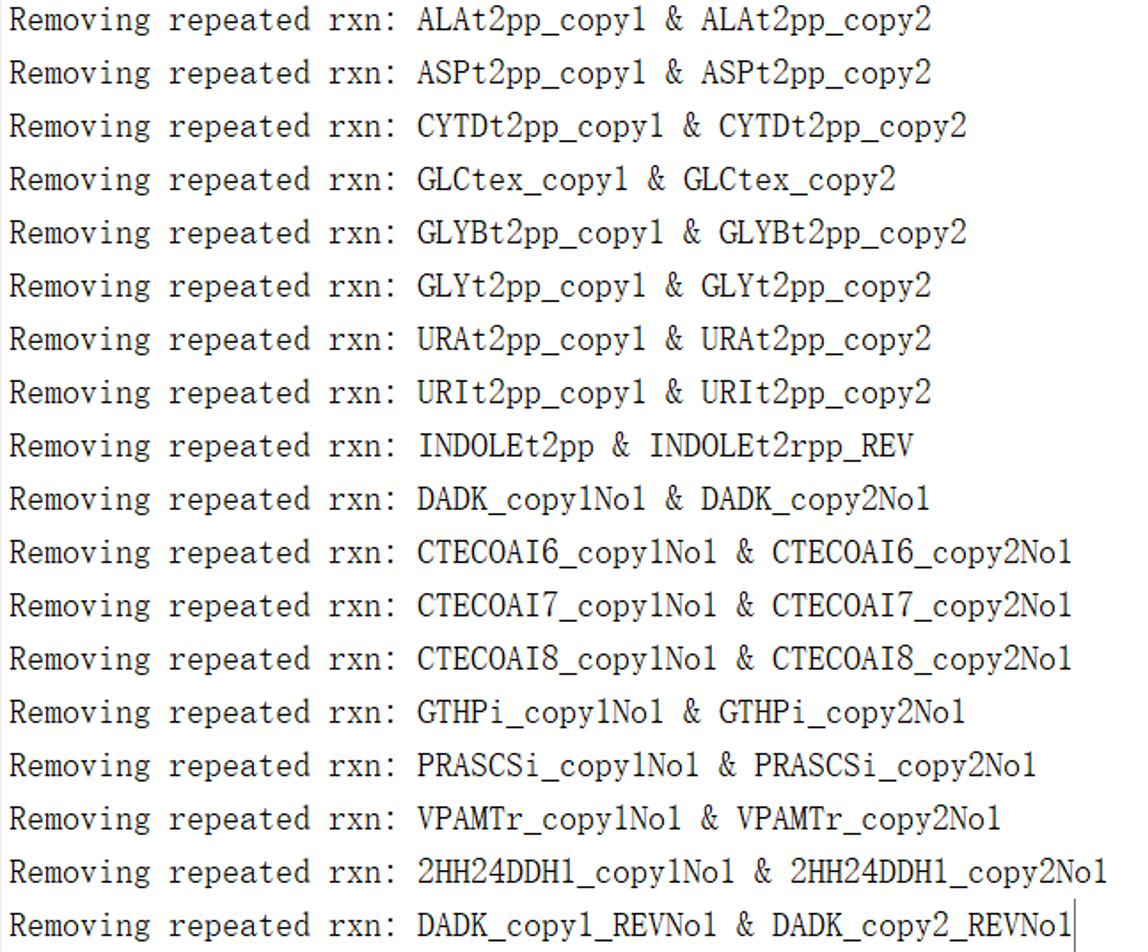Fig. 5. The modification results of ecModel

## Matching of enzyme constraint models to experimental data:

###### sigma    = 0.5;     %Optimized for glucose Ptot      = 0.55;    %Assumed constantgR_exp   = 0.77;    %[g/gDw h] Max batch gRate on minimal glucose media c_source = 'D-Glucose exchange (reversible)'; % Rxn name for the glucose uptake reaction. [ecModel_batch,OptSigma] = getConstrainedModel(ecModel,c_source,sigma,Ptot,gR_exp,modifications,name) (Fig. 6)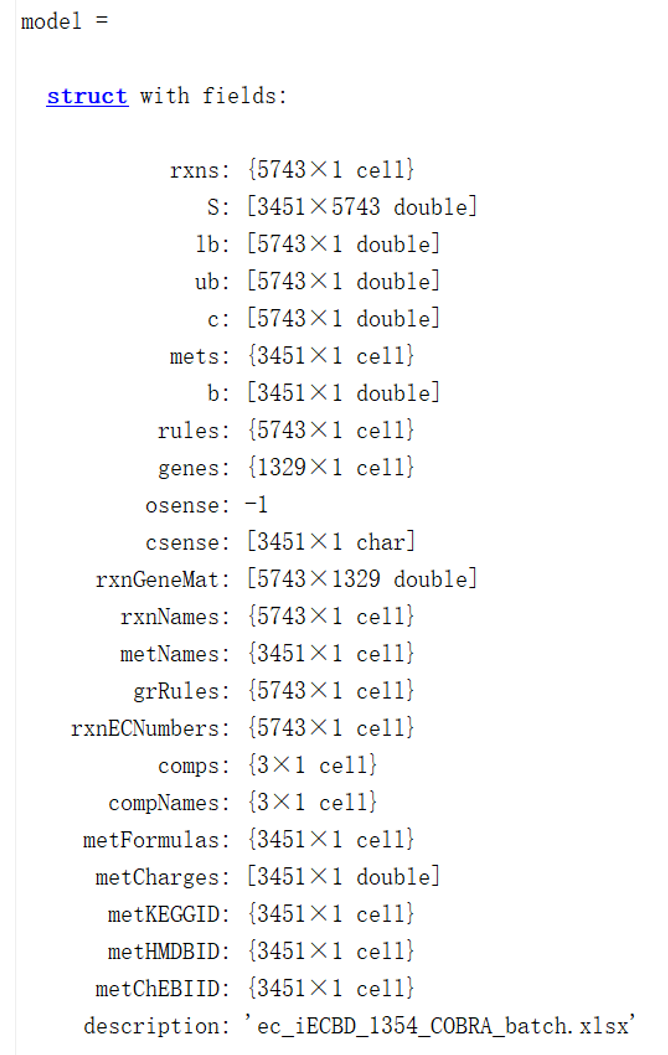Fig. 6. The final structure of model ec_iECBD_1354

## Analysis of properties of the enzyme-constrained model

###### Detailed information about ec_iECBD_1354 was listed in Table 2.
Table 2. The basic properties of enzyme-constrained model, ec_ iECBD_1354
General descriptors of the model
Number of reactions 5743
Number of metabolites 3451
Number of compartments 3
Classification of reactions
Metabolic reactions matched with an enzyme(s) 4721
Metabolic reactions not matched with an enzyme 1022
Transport reactions 1177
Metabolite exchange reactions 767
Arm reactions introduced for isozymes 384
Enzyme usages (treated as reactions) 632
Classification of metabolites
Original metabolites 1962
Enzymes 1105
Pseudo-metabolites introduced for isozymes 384
Enzyme/reaction relationships
Complexes 473
Reactions with isozymes 964
Reaction with single enzyme 3284
Promiscuous enzymes 16

## The verification of enzyme-constrained model

###### To verify the accuracy of enzyme-constrained model ec_iECBD_1354, we calculated the ability of using 14 kinds of carbon sources. Compared with other methods, the Pearson correlation coefficient (PCC) of the enzyme constraint model is 0.65 (p-value = 0.012), which is higher than other methods, except the MOMENT method (Fig. 7).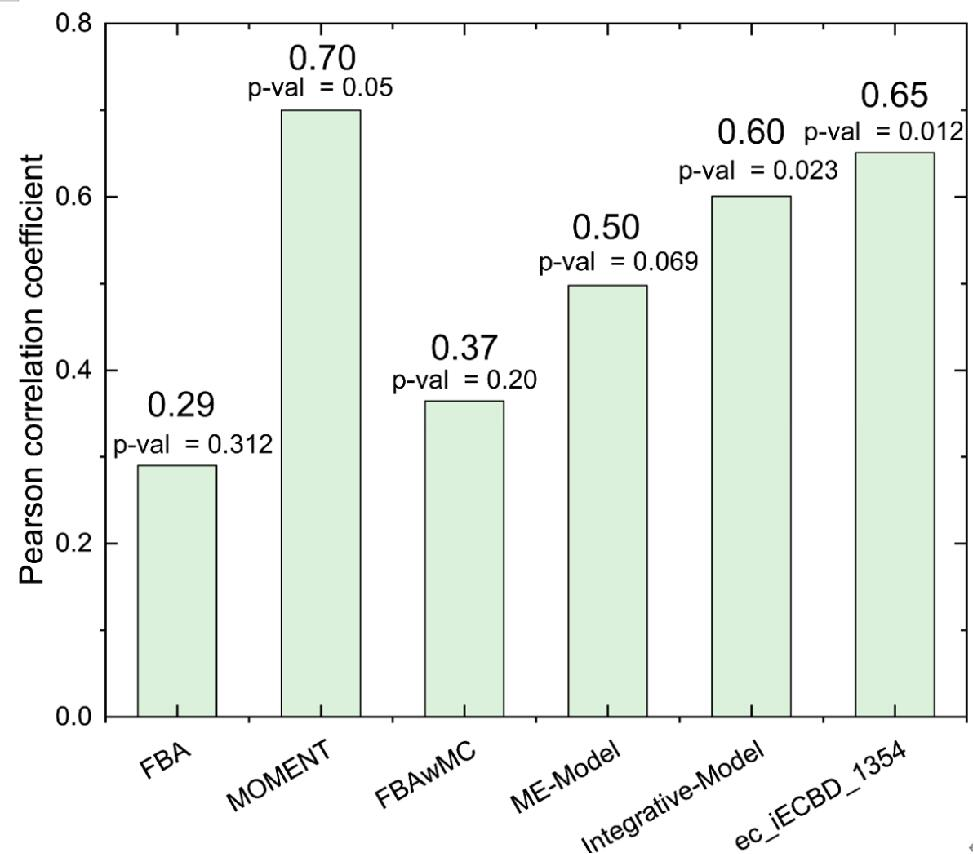Fig. 7. Comparison of the prediction accuracy among different enzymic constrains methods
###### In addition, we simulated consumption or production rate under different dilution rates, and the results were good agreement with experimental data (Fig. 8).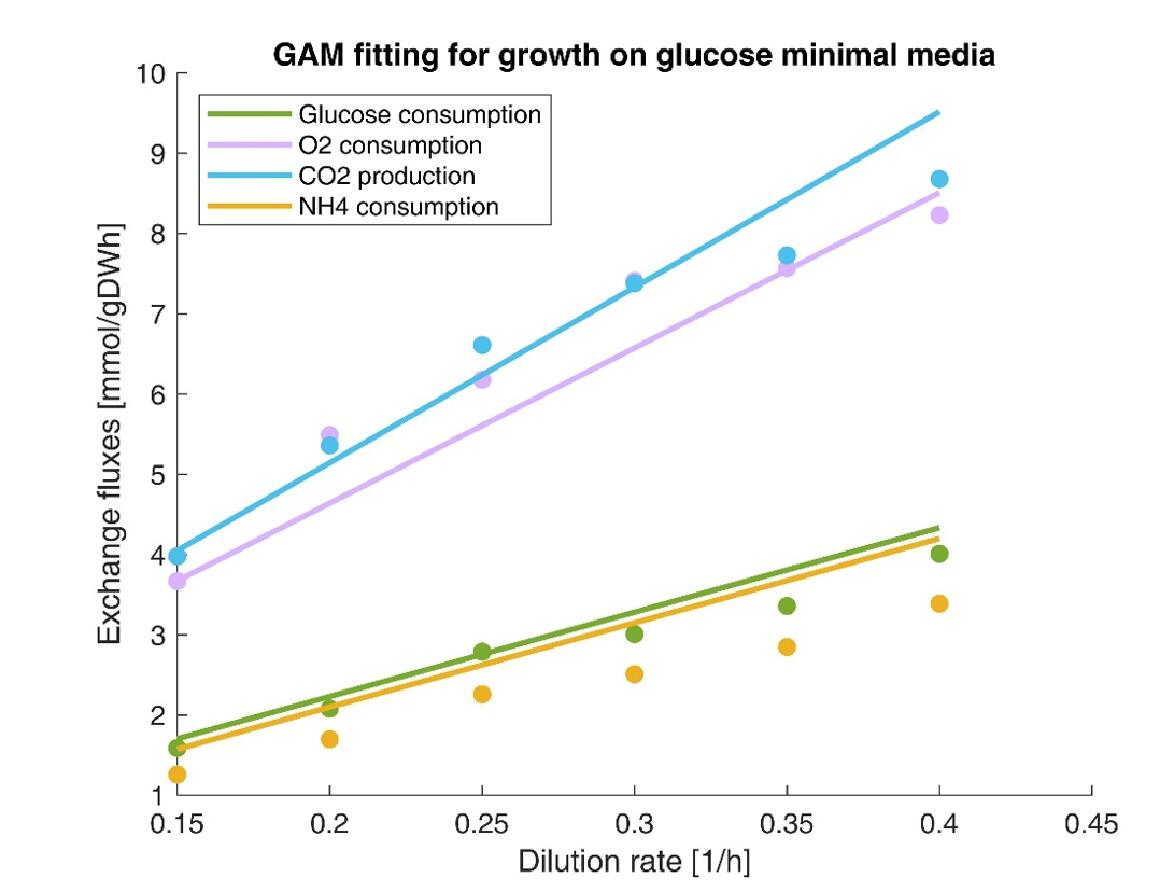Fig. 8. Comparison of the chemostat data with simulation results under different dilution rates. The lines represent simulation results and dots represent experimental data.

## Application of enzyme-constrained models:

###### After the verification of model ec_iECBD_1354, we further used it to identify the targets, which can improve the synthesis of SMAP protein. When comparing the differences of protein demand between cell growth stage and product synthesis stage, 7 proteins were identified as top-demand, which need to be up-regulated. While 21 proteins were classified as defined as reduced demand, which need to be down-regulated (Fig. 9, Table 3, Table 4).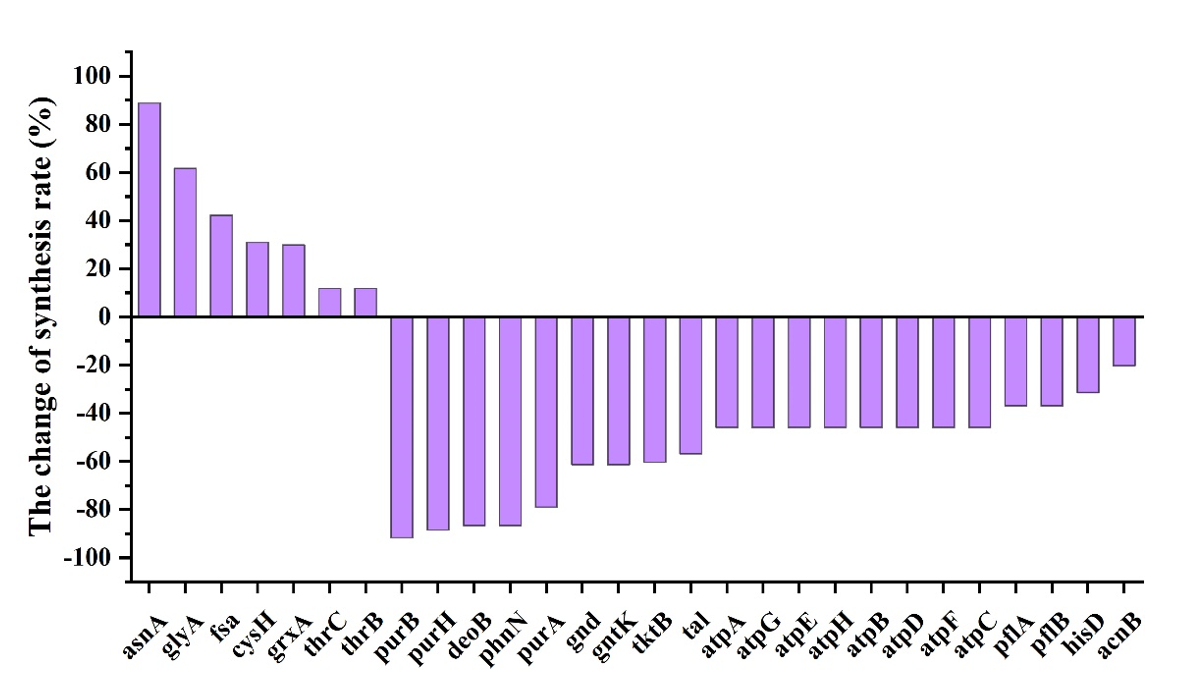Fig. 9. The demand of proteins need to be changed for SMAP synthesis.

Table 3. Proteins that increase in demand
Gene Name Function Reaction changes%
ECBD_4288 asnA Aspartate--ammonia ligase atp[c] + nh4[c] + asp__L[c] -> h[c] + ppi[c] + amp[c] + asn__L[c] 88.83
ECBD_1133 glyA Serine hydroxymethyltransferase h2o[c] + methf[c] -> h[c] + 5fthf[c] h2o[c] + methf[c] -> h[c] + 5fthf[c] 61.75
fsa Fructose-6-phosphate aldolase f6p[c] <=> g3p[c] + dha[c] 42.25
ECBD_0967 cysH Phosphoadenosine phosphosulfate reductase paps[c] + grxrd[c] -> 2 h[c] + pap[c] + so3[c] + grxox[c]1 31.0
ECBD_0115 grxA Glutaredoxin 29.96
ECBD_3614 thrC Threonine synthase h2o[c] + phom[c] -> pi[c] + thr__L[c] 11.88
ECBD_3615 thrB Homoserine kinase atp[c] + 4hthr[c] -> adp[c] + h[c] + phthr[c] 11.88

Table 4. Proteins that decrease in demand
Gene Name Function Reaction changes%
ECBD_2468 purB Adenylosuccinate lyase dcamp[c] <=> amp[c] + fum[c] -91.69
ECBD_4026 purH Bifunctional purine biosynthesis protein aicar[c] + 10fthf[c] <=> thf[c] + fprica[c] -88.53
ECBD_3637 deoB Phosphopentomutase r1p[c] <=> r5p[c] -86.68
ECBD_3936 phnN Ribose 1,5-bisphosphate phosphokinase atp[c] + r15bp[c] -> adp[c] + prpp[c] -86.66
ECBD_3857 purA Adenylosuccinate synthetase gtp[c] + asp__L[c] + imp[c] -> pi[c] + 2 h[c] + gdp[c] + dcamp[c] -79.03
ECBD_1630 gnd 6-phosphogluconate dehydrogenase,decarboxylating nadp[c] + 6pgc[c] -> co2[c] + nadph[c] + ru5p__D[c] -61.27
ECBD_0306 gntK Gluconokinase atp[c] + glcn[c] -> adp[c] + h[c] + 6pgc[c] -61.27
ECBD_1225 tktB Transketolase e4p[c] + xu5p__D[c] <=> f6p[c] + g3p[c] -60.36
ECBD_3610 tal Transketolase g3p[c] + s7p[c] <=> f6p[c] + e4p[c] -56.89
ECBD_4298 atpA ATP synthase subunit alpha adp[c] + pi[c] + 4 h[p] <=> atp[c] + h2o[c] + 3 h[c] -45.90
ECBD_4299 atpG ATP synthase gamma chain adp[c] + pi[c] + 4 h[p] <=> atp[c] + h2o[c] + 3 h[c] -45.90
ECBD_4295 atpE ATP synthase subunit c adp[c] + pi[c] + 4 h[p] <=> atp[c] + h2o[c] + 3 h[c] -45.90
ECBD_4297 atpH ATP synthase subunit delta adp[c] + pi[c] + 4 h[p] <=> atp[c] + h2o[c] + 3 h[c] -45.90
ECBD_4294 atpB ATP synthase subunit a adp[c] + pi[c] + 4 h[p] <=> atp[c] + h2o[c] + 3 h[c] -45.90
ECBD_4300 atpD ATP synthase subunit beta adp[c] + pi[c] + 4 h[p] <=> atp[c] + h2o[c] + 3 h[c] -45.90
ECBD_4296 atpF ATP synthase subunit b adp[c] + pi[c] + 4 h[p] <=> atp[c] + h2o[c] + 3 h[c] -45.90
ECBD_4301 atpC ATP synthase epsilon chain adp[c] + pi[c] + 4 h[p] <=> atp[c] + h2o[c] + 3 h[c] -45.90
ECBD_2692 pflA Formate C-acetyltransferase coa[c] + 2obut[c] -> ppcoa[c] + for[c] -36.97
ECBD_2693 pflB Pyruvate formate-lyase-activating enzyme coa[c] + pyr[c] -> accoa[c] + for[c] -36.97
ECBD_1639 hisD Histidinol dehydrogenase h2o[c] + 2 nad[c] + histd[c] -> 3 h[c] + 2 nadh[c] + his__L[c] -31.41
ECBD_3501 acnB Aconitate hydratase B cit[c] <=> h2o[c] + acon_C[c] -20.34

## Application of enzyme-constrained models:

###### In order to test the effective of target genes, we selected the asnA and purA from the 21 proteins. Furthermore, the asnA gene was overexpressed with the strong promoter of Pj23119 at the IS site in the genome of BL21 (DE3), yielding the engineered strain of asnA. And, the purA gene was knocked out from the genome of BL21(DE3), yielding the engineered strain of △purA. Firstly, we tested the growth of two engineered strains when no AMP was expressed. Results showed that two engineered strains all keep the similar growth with the wild strain (Fig. 10).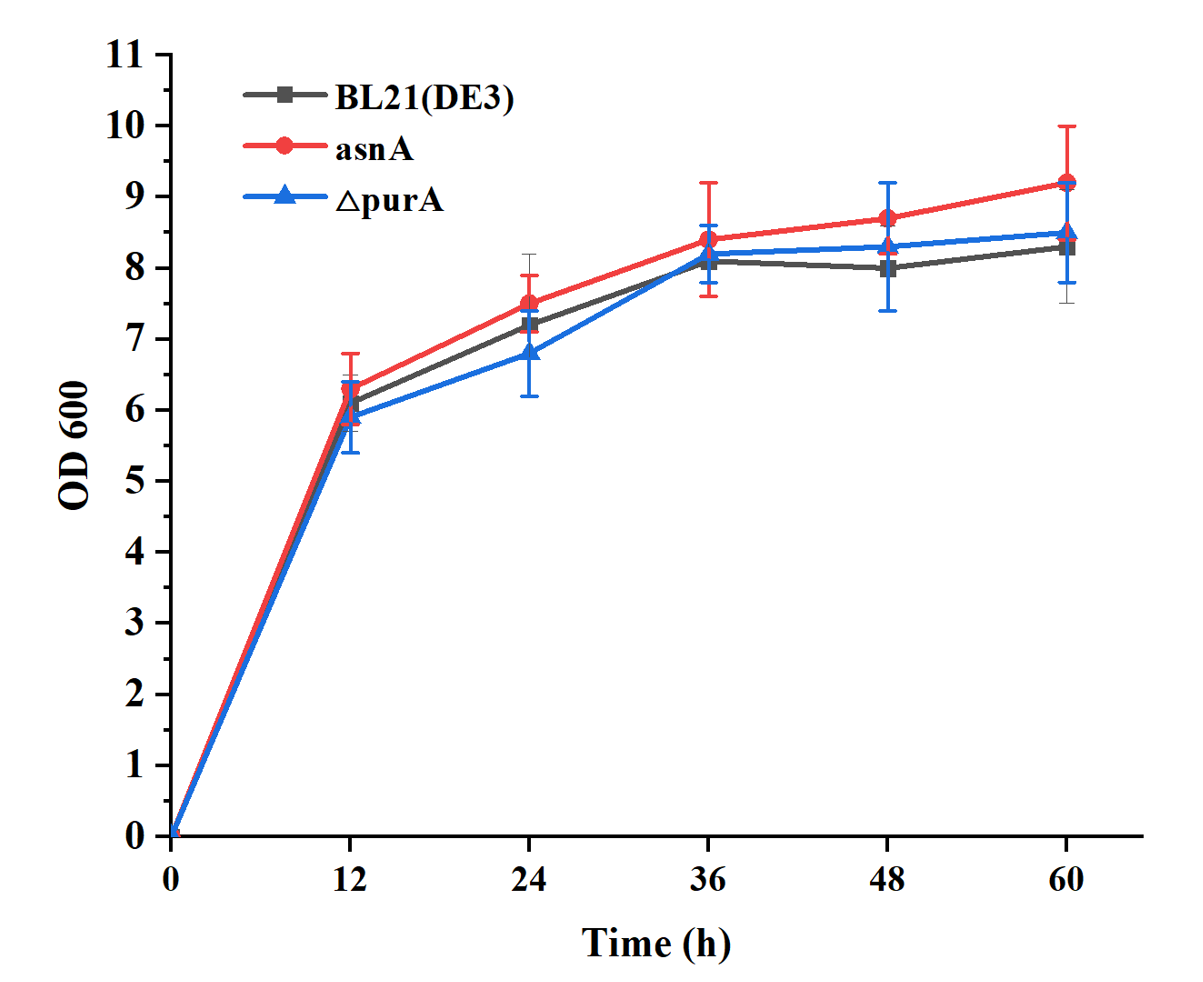Fig. 10. The OD600 of the BL21(DE3), asnA and △purA strain.
###### Furthermore, engineering strains asnA and △purA were tested for the expression of AMPs. When overexpressed the AMPs of SFa and SMAP, two engineered strains showed advantages over the wild strain (Fig. 11). The expression of SFa was increased by 53.5% and 150% in asnA and △purA strains, respectively. The expression of SMAP was increased by89% and 63.3%, respectively. These results show that asnA and purA gene can be used to further regulate the production of AMPs, and which also prove that the model has certain accuracy and reference value, which can provide prediction for improving the production of AMPs.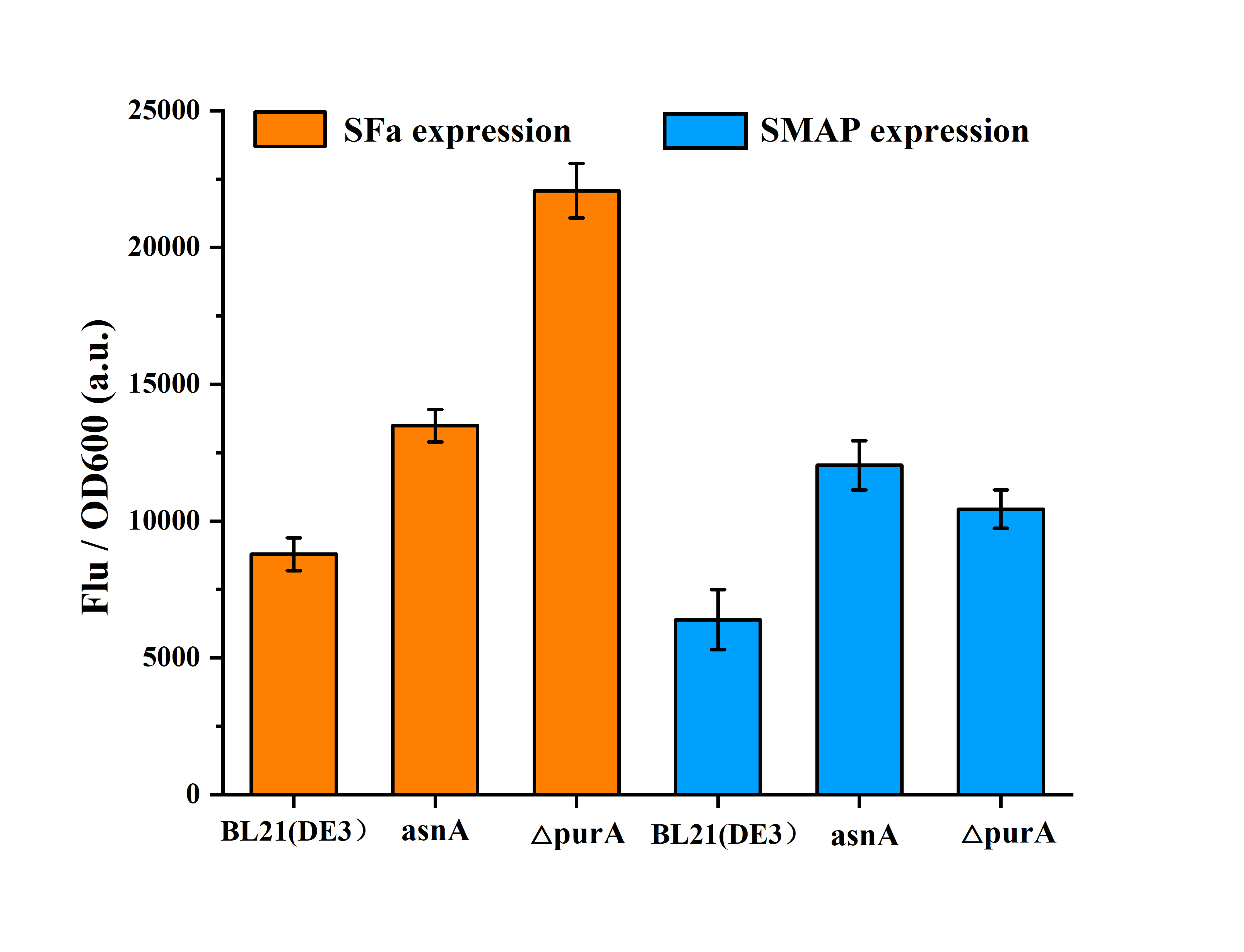Fig. 11. The sFa and SMAP expression in the BL21 (DE3), asnA and △purA strain
References

 Paalme, T., Elken, R., Kahru, A. et al. The growth rate control in Escherichia coli at near to maximum growth rates: the A-stat approach. Antonie Van Leeuwenhoek 71, 217–230 (1997).
.  Thiele, I.,Palsson, B. Ø. A protocol for generating a high-quality genome-scale metabolic reconstruction[J]. Nat. Protoc., 2010, 5 (1): 93-121.
.  Sanchez, B. J.,Zhang, C.,Nilsson, A.,Lahtvee, P. J.,Kerkhoven, E. J.,Nielsen, J. Improving the phenotype predictions of a yeast genome-scale metabolic model by incorporating enzymatic constraints[J]. Mol. Syst. Biol., Aug 3, 2017, 13 (8): 935.